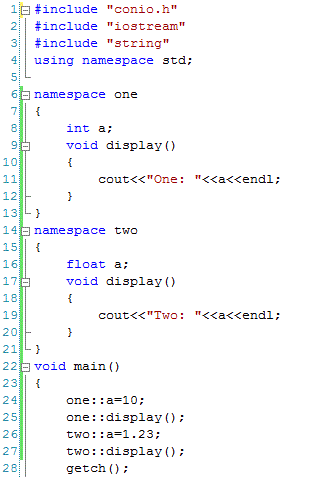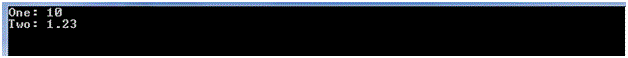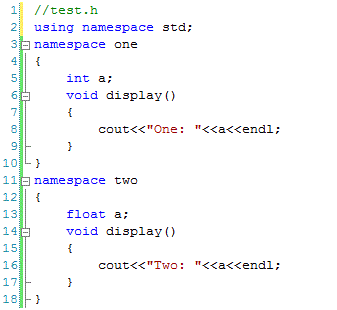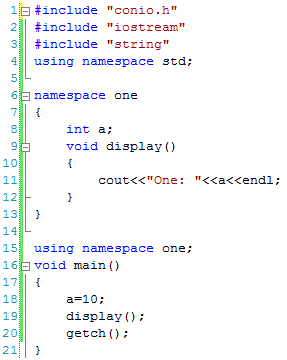# Namespaces in C++

## Namespaces in C++ and How to use them

Namespace can be considered as a container where identifiers including variables, classes or functions are declared or defined. Namespaces are useful when different identifiers having same names are defined having different meanings in different contexts.

For instance an identifier ‘address’ can be declared in one namespace to represent ‘home address’ while in another namespace to represent ‘office address’. It is important to note that one namespace can be used in more than one programs and one program can use more than one namespaces simultaneously.

Defining and Using Namespaces

If we are required to use the same variable and function differently but in the same program then we can do it with the help of example as shown below.We can see that variable ‘a’ and function ‘display’ are defined both in namespace one and namespace two and being used in main function. If we compile and run the code then the result will as below.Using Same Namespace in Multiple Programs

A powerful feature of namespaces is that they are defined once and used in more than one files. it allows us to write the code once and use more than one time in different programs. For instance we can save the following code in a file test.h and use it in a C++ program by including the header file as shown below.Save the above code in a file test.h and include it in a C++ program as below.We can see that test.h file having namespaces is included at line 4. In main function we can use all the identifiers defined in either namespace one or in namespace two without defining them again.

Use of Using Directive

The scope resolution operator (::) is not required to be used if we use ‘using’ directive as shown below.We can see that once namespace one is used at line 15 then there is no need to refer to identifiers of this namespace by using scope resolution operator (::) at line 18 and 19. However the problem with this approach is that we cannot define the same identifier in more than one namespaces. If used then it will refer to the identifier of namespace called by using directive. For example, consider the following code.If we compile this code, it will raise errors at line 25, 26, 27 and 28 as these identifiers are defined both in namespace one and namespace two. Compiler will not be able to understand that whether identifiers of namespace one are referred in these lines or identifiers of namespace two. In such situations these lines should be written as below.

void main()

{

one::a=10;

one::display();

two::a=1.23;

two::display();

getch();

}